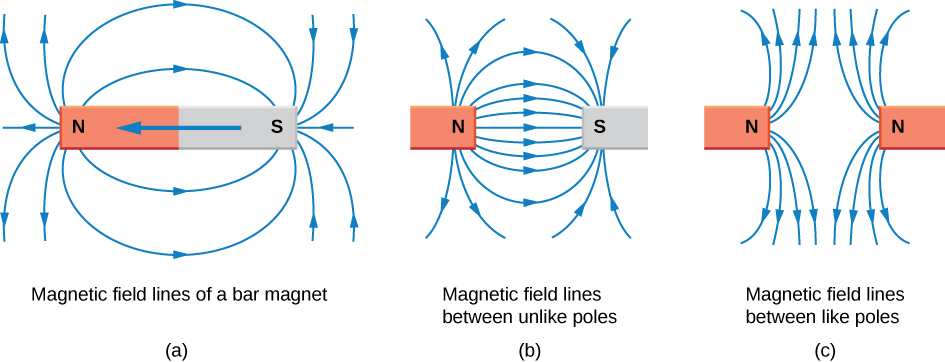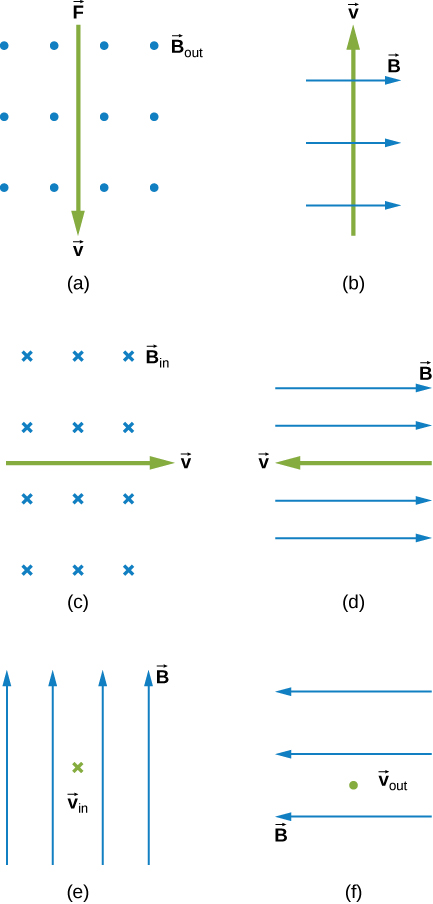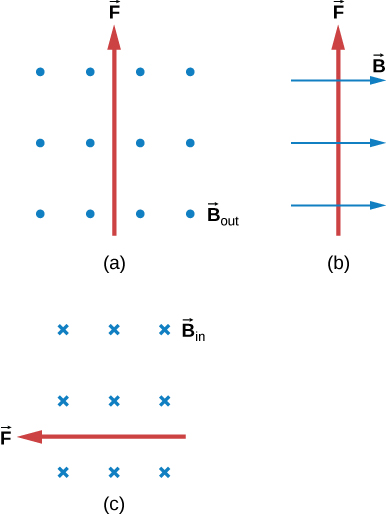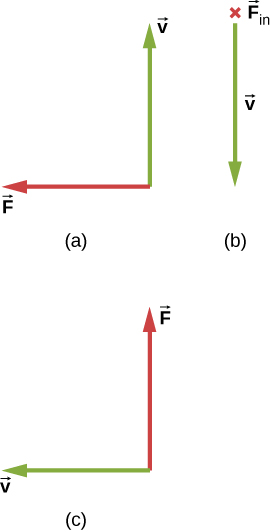# 11.2 Magnetic fields and lines  (Page 3/8)

 Page 3 / 8

## Significance

The cross product in this formula results in a third vector that must be perpendicular to the other two. Other physical quantities, such as angular momentum, also have three vectors that are related by the cross product. Note that typical force values in magnetic force problems are much larger than the gravitational force. Therefore, for an isolated charge, the magnetic force is the dominant force governing the charge’s motion.

Check Your Understanding Repeat the previous problem with the magnetic field in the x -direction rather than in the z -direction. Check your answers with RHR-1.

a. 0 N; b. $2.4\phantom{\rule{0.2em}{0ex}}×\phantom{\rule{0.2em}{0ex}}{10}^{-14}\stackrel{^}{k}\text{N};$ c. $2.4\phantom{\rule{0.2em}{0ex}}×\phantom{\rule{0.2em}{0ex}}{10}^{-14}\stackrel{^}{j}\phantom{\rule{0.2em}{0ex}}\text{N};$ d. $\left(7.2\stackrel{^}{j}+2.2\stackrel{^}{k}\right)\phantom{\rule{0.2em}{0ex}}×\phantom{\rule{0.2em}{0ex}}{10}^{-15}\text{N}$

## Representing magnetic fields

The representation of magnetic fields by magnetic field lines    is very useful in visualizing the strength and direction of the magnetic field. As shown in [link] , each of these lines forms a closed loop, even if not shown by the constraints of the space available for the figure. The field lines emerge from the north pole (N), loop around to the south pole (S), and continue through the bar magnet back to the north pole.

Magnetic field lines have several hard-and-fast rules:

1. The direction of the magnetic field is tangent to the field line at any point in space. A small compass will point in the direction of the field line.
2. The strength of the field is proportional to the closeness of the lines. It is exactly proportional to the number of lines per unit area perpendicular to the lines (called the areal density).
3. Magnetic field lines can never cross, meaning that the field is unique at any point in space.
4. Magnetic field lines are continuous, forming closed loops without a beginning or end. They are directed from the north pole to the south pole.

The last property is related to the fact that the north and south poles cannot be separated. It is a distinct difference from electric field lines, which generally begin on positive charges and end on negative charges or at infinity. If isolated magnetic charges (referred to as magnetic monopoles ) existed, then magnetic field lines would begin and end on them.Magnetic field lines are defined to have the direction in which a small compass points when placed at a location in the field. The strength of the field is proportional to the closeness (or density) of the lines. If the interior of the magnet could be probed, the field lines would be found to form continuous, closed loops. To fit in a reasonable space, some of these drawings may not show the closing of the loops; however, if enough space were provided, the loops would be closed.

## Summary

• Charges moving across a magnetic field experience a force determined by $\stackrel{\to }{F}=q\stackrel{\to }{v}\phantom{\rule{0.2em}{0ex}}×\phantom{\rule{0.2em}{0ex}}\stackrel{\to }{B}.$ The force is perpendicular to the plane formed by $\stackrel{\to }{v}$ and $\stackrel{\to }{B}.$
• The direction of the force on a moving charge is given by the right hand rule 1 (RHR-1): Sweep your fingers in a velocity, magnetic field plane. Start by pointing them in the direction of velocity and sweep towards the magnetic field. Your thumb points in the direction of the magnetic force for positive charges.
• Magnetic fields can be pictorially represented by magnetic field lines, which have the following properties:
1. The field is tangent to the magnetic field line.
2. Field strength is proportional to the line density.
3. Field lines cannot cross.
4. Field lines form continuous, closed loops.
• Magnetic poles always occur in pairs of north and south—it is not possible to isolate north and south poles.

## Conceptual questions

Discuss the similarities and differences between the electrical force on a charge and the magnetic force on a charge.

Both are field dependent. Electrical force is dependent on charge, whereas magnetic force is dependent on current or rate of charge flow.

(a) Is it possible for the magnetic force on a charge moving in a magnetic field to be zero? (b) Is it possible for the electric force on a charge moving in an electric field to be zero? (c) Is it possible for the resultant of the electric and magnetic forces on a charge moving simultaneously through both fields to be zero?

## Problems

What is the direction of the magnetic force on a positive charge that moves as shown in each of the six cases?a. left; b. into the page; c. up the page; d. no force; e. right; f. down

Repeat previous exercise for a negative charge.

What is the direction of the velocity of a negative charge that experiences the magnetic force shown in each of the three cases, assuming it moves perpendicular to B ?a. right; b. into the page; c. down

Repeat previous exercise for a positive charge.

What is the direction of the magnetic field that produces the magnetic force on a positive charge as shown in each of the three cases, assuming $\stackrel{\to }{B}$ is perpendicular to $\stackrel{\to }{v}$ ?a. into the page; b. left; c. out of the page

Repeat previous exercise for a negative charge.

(a) Aircraft sometimes acquire small static charges. Suppose a supersonic jet has a 0.500-μC charge and flies due west at a speed of 660. m/s over Earth’s south magnetic pole, where the $8.00\phantom{\rule{0.2em}{0ex}}×\phantom{\rule{0.2em}{0ex}}{10}^{-5}-\text{T}$ magnetic field points straight up. What are the direction and the magnitude of the magnetic force on the plane? (b) Discuss whether the value obtained in part (a) implies this is a significant or negligible effect.

a. $2.64\phantom{\rule{0.2em}{0ex}}×\phantom{\rule{0.2em}{0ex}}{10}^{-8}\phantom{\rule{0.2em}{0ex}}\text{N};$ b. The force is very small, so this implies that the effect of static charges on airplanes is negligible.

(a) A cosmic ray proton moving toward Earth at $5.00\phantom{\rule{0.2em}{0ex}}×\phantom{\rule{0.2em}{0ex}}{10}^{7}\text{m/s}$ experiences a magnetic force of $1.70\phantom{\rule{0.2em}{0ex}}×\phantom{\rule{0.2em}{0ex}}{10}^{-16}\phantom{\rule{0.2em}{0ex}}\text{N}.$ What is the strength of the magnetic field if there is a 45º angle between it and the proton’s velocity? (b) Is the value obtained in part a. consistent with the known strength of Earth’s magnetic field on its surface? Discuss.

An electron moving at $4.00\phantom{\rule{0.2em}{0ex}}×\phantom{\rule{0.2em}{0ex}}{10}^{3}\text{m/s}$ in a 1.25-T magnetic field experiences a magnetic force of $1.40\phantom{\rule{0.2em}{0ex}}×\phantom{\rule{0.2em}{0ex}}{10}^{-16}\phantom{\rule{0.2em}{0ex}}\text{N}.$ What angle does the velocity of the electron make with the magnetic field? There are two answers.

$10.1\text{°};169.9\text{°}$

(a) A physicist performing a sensitive measurement wants to limit the magnetic force on a moving charge in her equipment to less than $1.00\phantom{\rule{0.2em}{0ex}}×\phantom{\rule{0.2em}{0ex}}{10}^{-12}\phantom{\rule{0.2em}{0ex}}\text{N}.$ What is the greatest the charge can be if it moves at a maximum speed of 30.0 m/s in Earth’s field? (b) Discuss whether it would be difficult to limit the charge to less than the value found in (a) by comparing it with typical static electricity and noting that static is often absent.

Figure, shows a pencil partially immersed in a cup of water. Why does the pencil appear to be ?bent
what is heat capacity
joule
defined as the amount of heat to be supplied to an object to produce a unit change in its temperature. The SI unit of heat capacity is joule per kelvin
formula of acid hydride
what is mass
mass is the amount of staff made up an object
Van
physic is anatural science that deals about scleral
Abdilahi
mass is accelaration divided force
Abdilahi
mass is force divided by acceleration
Van
Is the amout of matter contain in a body
what is the volume of a human being
Why does the lines of force not touch each other 🇲🇲
what is physics
Exildah
physics is the study of matter and energy and the interaction between them
Van
what is unit
Please canu get more questions on electric field and electric flux please
Gbemisola
is electric field directly proportional to the squared of a distance
No electric field is inversely proportional to the squared distance between the charges
Gbemisola
The bullet 2.00cm long is fired at 420/s and passes straight through a 10.0 cm thick board existing at 280 m/s.What is the average acceleration of the bullet through the board?
FAUSTINA
an unstretched spring is 12cm long .A load of 5N stretched it to 15cm .how long will it be under a load of 15N?
Morapeli
hi
Africa
hi
Benjamin
Benjamin how are u are u a freshman in the university
Africa
like 100 level
Africa
yes sir
Benjamin
l need a physics tutor
Benjamin
I think the best tutor is God and organic tutor in YouTube that guy is good
Africa
me too 100level
Africa
wow nice
Benjamin
from Nigeria and u
Africa
I am from Nigeria and u wow nice that something I use to always say
Africa
am from Ghana
Benjamin
ok
Africa
studying what
Africa
Compare the electric flux through the surface of a cube of side length a that has a charge q at its center to the flux through a spherical surface of radius a with a charge q at its center.
please I want to know how to solve increase in length
Ujah
Why a charged capacitor has potential difference but not emf
what is the dimension symbol of temperature?
what is the dimension symbol of temperature?
Keren
what's the meaning of enthalpy in terms of latent heat, internal energy, phase change
Enthalpy is the degree ofdisorderlinessof a substance
Gbemisola
how to convert Kelvin to centigrade
what is the s, p, d, f in this table
Sangeetha
s, p, d, f in this table
Sangeetha
what kind of table this
Vengata
Periodic table
Gbemisola
what are waves
In physics, mathematics, and related fields, a wave is a propagating dynamic disturbance (change from equilibrium) of one or more quantities

#### Get Jobilize Job Search Mobile App in your pocket Now!By Steve GibbsBy Stephen VoronByBy Jessica CollettBy OpenStaxBy Jonathan LongBy Ellie BanfieldBy Brooke DelaneyBy Jonathan LongBy OpenStax